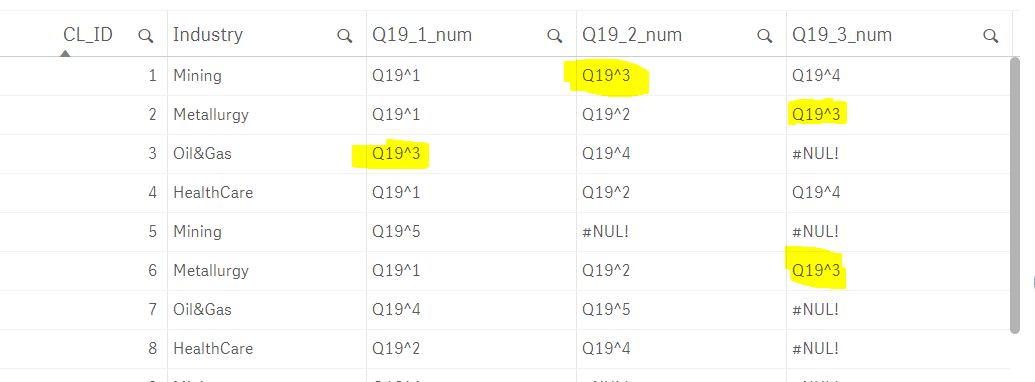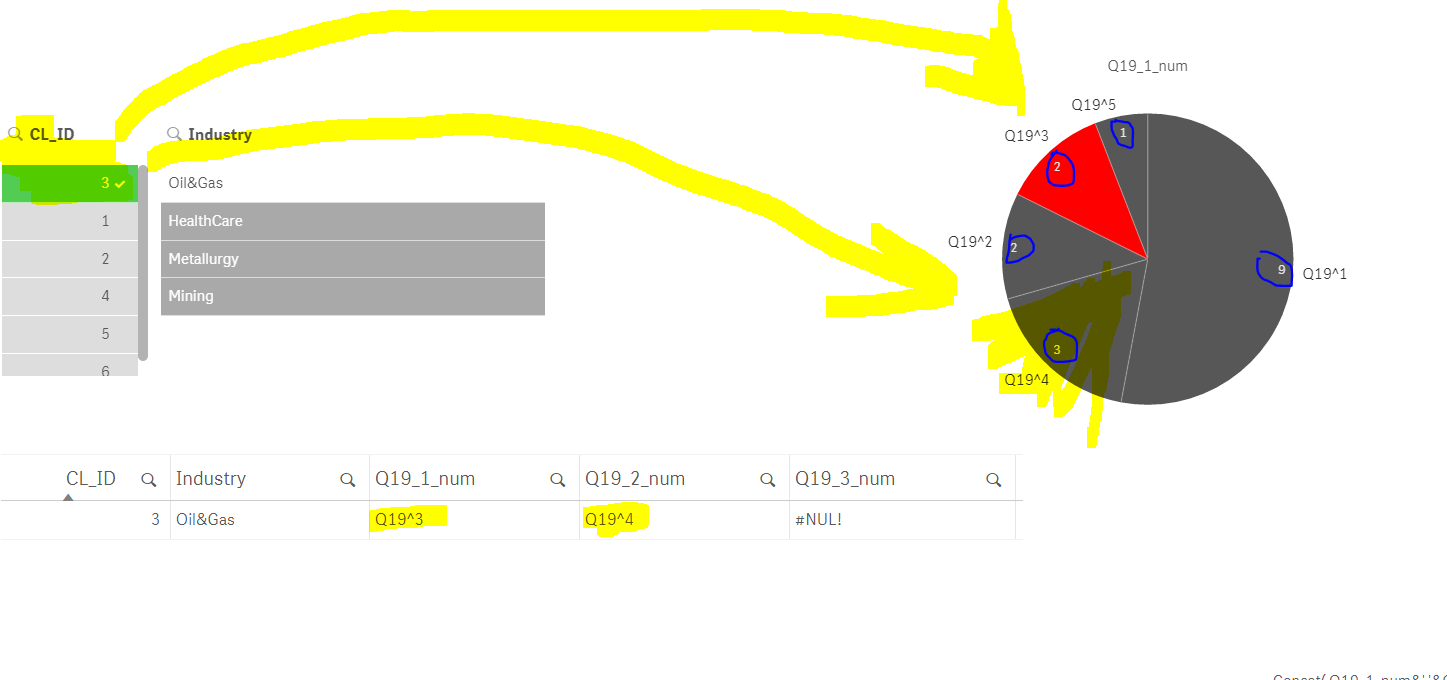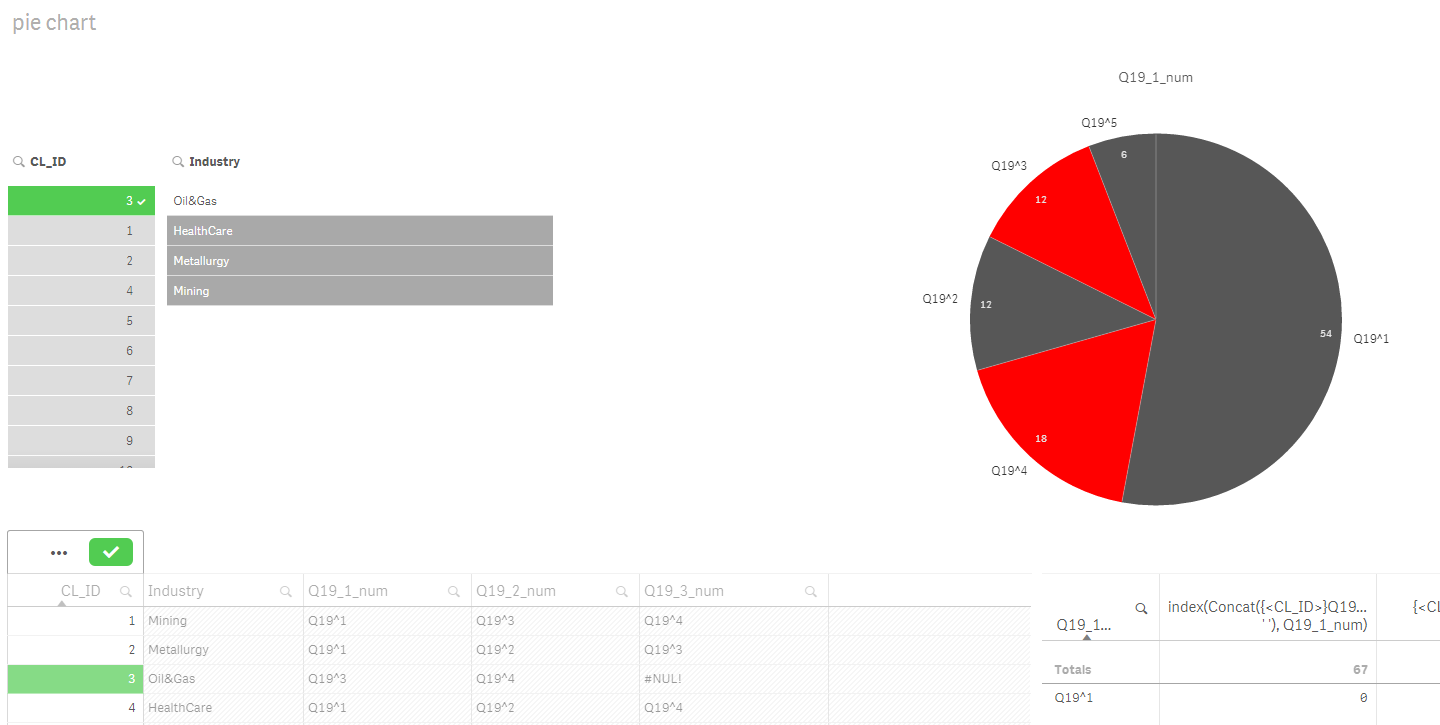# New to Qlik Sense

Discussion board where members can get started with Qlik Sense.

Announcements
Qlik® Product Spotlight: Discover what’s possible. Get more from our products.
See for yourself. Register today.
Not applicable

## Highlight a pie chart by expression - with complications

Hi all!

I'm creating a comparison of each client answer vs all answers.

I have a client answer data below:

multiple answers to the question are possible and they are given in several (6, here shown 3) columns.:When I select one CL_ID, I need to see the pie chart with:

1. all answers of that CL_ID highlighted (now I see only first column highlighted),

2. on pie chart I need to see total numbers (count) of each answer (now I see only totals from first field only)So, the data is in 6 columns, and I need to show

1) all of it in 1 pie chart

2) with ability to show count for answers from all 6 columns

3) and highlight the regions which are presented in chosen CL_ID row (when 1 CL_ID is selected).

File with example is attached...

Can you help me, please?Tags (7)
4 Replies
Not applicable

## Re: Highlight a pie chart by expression - with complications

Check the attached maybeColor Expression:

If(Len(GetCurrentSelections())>0

and (index(Concat(DISTINCT {<CL_ID>}Q19_1_num, ' '), Only(TOTAL Q19_1_num)) >=1  or

index(Concat(DISTINCT {<CL_ID>}Q19_1_num, ' '), Only(TOTAL Q19_2_num)) >=1  or

index(Concat(DISTINCT {<CL_ID>}Q19_1_num, ' '), Only(TOTAL Q19_3_num)) >=1  or

index(Concat(DISTINCT {<CL_ID>}Q19_1_num, ' '), Only(TOTAL Q19_4_num)) >=1  or

index(Concat(DISTINCT {<CL_ID>}Q19_1_num, ' '), Only(TOTAL Q19_5_num)) >=1  or

index(Concat(DISTINCT {<CL_ID>}Q19_1_num, ' '), Only(TOTAL Q19_6_num)) >=1

)

, rgb(255, 0, 0), rgb(87, 87, 87))

Measure

RangeSum(

Count({<CL_ID=>}Q19_1_num),

Count({<CL_ID=>}Q19_2_num),

Count({<CL_ID=>}Q19_3_num),

Count({<CL_ID=>}Q19_4_num),

Count({<CL_ID=>}Q19_5_num),

Count({<CL_ID=>}Q19_6_num))

Not applicable

## Re: Highlight a pie chart by expression - with complications

Yes, you highlight it correctly.

But the total numbers (measure count) of each answer (Q19^1, Q19^2 etc) on pie chart are incorrect.The data used in example has only:and on the pie chart we see 54 of Q19^1, but actually it should be 9

So please make it count totals right...

Not applicable

## Re: Highlight a pie chart by expression - with complications

The way it is currently structured, I don't think this would be possible.... May be use a new island dimension to do this....

Not applicable

## Re: Highlight a pie chart by expression - with complications

What I cannot do is to alter the huge  table with answers (inputs) - loaded inline in this example.

If you know how to make it work with new dimension using script - be so kind to show it....Community Browser